# Bonded Blades in CompressionThis test lets you check the bending/extension coupling in non-symmetric stack composite plates and the traverse shear behavior in a thick plate.

For this test, the reference is the mesh formed of linear hexahedron elements (HE8). You will use 2D meshes.Reference:

3D Model with HE8 element, computed with CATIA.

##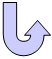Specifications

### Geometry Specifications

 Length: L = 10 000 mm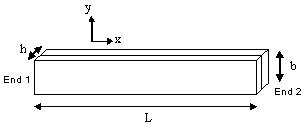Width: b = 1 000 mm Thickness: h = 400 mm divided in two layers

### Analysis Specifications

 Material 1: Young Modulus (material): E = 100 MPa Poisson's Ratio: ν = 0.3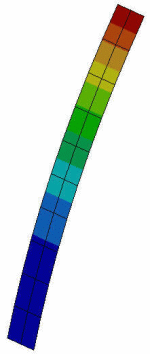Material 2: Young Modulus (material): E = 10 MPa Poisson's Ratio: ν = 0.2 Mesh Specifications: 10 x 2 elements for linear quadrangle (QD4) and parabolic quadrangle (QD8) elements 10 x 2 x 1 elements for linear hexahedron elements (HE8) Restraints: Clamp on End 1 Loads:Force density Fx = -30 N on End 2

##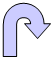Results

The static analysis results correspond to the displacements Tx and Tz at End 2.

The buckling analysis is performed for this 30 N load case. The buckling coefficients for the lowest two modes, computed with CATIA, are presented in the table below:

 Analysis Case Quantity Reference  Linear Hexahedron  (HE8) Computed Results Linear Quadrangle  (QD4) Parabolic Quadrangle (QD8)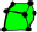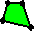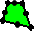Static Case Tx max [mm] -0.027 -0.0277 -0.0281 Tz max [mm] -0.834 -0.834 -0.835 Buckling Case Coefficient 1 121.634 121.197 120.743 Coefficient 2 1126.56 1095.34 1079.94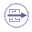To Perform the Test:

The Bonded_blades_compression_QD8.CATAnalysis document presents a complete analysis of this case, computed with a mesh formed of parabolic quadrangle elements (QD8).

The Bonded_blades_compression_HE8.CATAnalysis document presents a complete analysis of this case, computed with a mesh formed of linear hexahedron elements (HE8).

To compute the mesh with linear quadrangle elements (QD4), proceed as follow:

1. Open the Bonded_blades_compression_QD8.CATAnalysis document.

2. Enter the Advanced Meshing Tools workbench.

3. In the specification tree, double-click the mesh part. The Global Parameters dialog box appears.

4. Select Linear as element type.

5. Compute the case in the Generative Structural Analysis workbench.